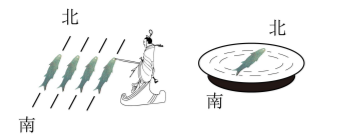$\text{A.}$ 鱼形铁不能被磁体吸引 $\text{B.}$ 指南鱼周围存在磁感线 $\text{C.}$ 指南鱼鱼头应标注 “ $\mathrm{N}$ ” $\text{D.}$ 指南鱼的腹部磁性最强